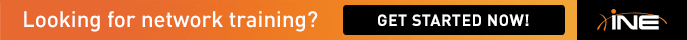# BB1 S0/0 Won't come up

Guys,

guys,

i wondered if you could help me out.

i am trying to establish connectivity through the ine topology and for some reason the serial0/0 will not come up across the PVC to R6. The Frame Relay
map statements are all showing inactive, i have a number of debug, the only one that really shows any decent is that i get " DCE LMI timeout" on BB1

The configuration is below,

BB1
!
frame-relay switching
!
interface Serial0/0
description Connected to R6
encapsulation frame-relay
clockrate 64000
frame-relay intf-type dce
no shutdown
!
interface Serial0/0.101 point-to-point
description PVC 101 to R6
ip authentication mode eigrp 10 md5
ip authentication key-chain eigrp 10 EIGRP
ipv6 rip RIPng enable
frame-relay interface-dlci 101
!

R6
!
interface Serial0/0
encapsulation frame-relay
clock rate 2000000
frame-relay map ip 54.1.1.254 101 broadcast
!

.NET FILE

##############################################################################################
#
# Internetwork Expert Routing & Switching topology Version 5.0 mappings for dynamips/GNS3 on Mac OS X
#
#
#  Dynamips: http://www.ipflow.utc.fr/index.php/Cisco_7200_Simulator
#  Dynagen:  http://dyna-gen.sourceforge.net/
#  GNS3: http://www.gns3.net
#  Hacki's Forum: http://hacki.at/7200emu/index.php
#  Internetwork Expert's Forum: http://IEOC.com
#
##############################################################################################

autostart = False
[localhost:7200]
udp = 10000
workingdir = C:GNS3Working
[]
image = C:Program FilesDynamipsimagesc3725-124.bin
ram = 128
ghostios = True
idlepc = 0x60a847cc
idlemax = 100
[[ROUTER R1]]
model = 3725
console = 2001
cnfg = C:DynamipsVol1Initial EIGRP
1.cfg
s0/0 = FR0 1
s0/1 = R3 s1/2
f0/0 = SW1 f1/1
x = -288.0
y = -53.0
[[ROUTER R2]]
model = 3725
console = 2002
cnfg = C:DynamipsVol1Initial EIGRP
2.cfg
s0/0 = FR0 2
s0/1 = R3 s1/3
f0/0 = SW2 f1/2
x = -648.0
y = -62.0
[[ROUTER R3]]
model = 3725
console = 2003
cnfg = C:DynamipsVol1Initial EIGRP
3.cfg
f0/0 = SW1 f1/3
f0/1 = SW3 f1/3
slot1 = NM-4T
s1/0 = FR0 3
s1/1 = FR0 4
s1/2 = R1 s0/1
s1/3 = R2 s0/1
x = -462.0
y = -102.0
[localhost:7201]
udp = 11000
workingdir = C:GNS3Working
[]
image = C:Program FilesDynamipsimagesc3725-124.bin
ram = 128
ghostios = True
idlepc = 0x60a847cc
idlemax = 100
[[ROUTER R4]]
model = 3725
console = 2004
cnfg = C:DynamipsVol1Initial EIGRP
4.cfg
s0/0 = FR0 5
s0/1 = R5 s0/1
f0/0 = SW2 f1/4
f0/1 = SW4 f1/4
x = -685.0
y = 90.0
[[ROUTER R5]]
model = 3725
console = 2005
cnfg = C:DynamipsVol1Initial EIGRP
5.cfg
s0/0 = FR0 6
s0/1 = R4 s0/1
f0/0 = SW1 f1/5
f0/1 = SW3 f1/5
x = -503.0
y = 256.0
[[ROUTER R6]]
model = 3725
console = 2006
cnfg = C:DynamipsVol1Initial EIGRP
6.cfg
s0/0 = BB1 s0/0
f0/0 = SW2 f1/6
f0/1 = SW4 f1/6
x = -336.0
y = 180.0
[[ROUTER SW1]]
model = 3725
console = 2007
cnfg = C:DynamipsVol1Initial EIGRPsw1.cfg
slot1 = NM-16ESW
f1/1 = R1 f0/0
f1/3 = R3 f0/0
f1/5 = R5 f0/0
f1/7 = SW2 f1/7
f1/8 = SW2 f1/8
f1/9 = SW2 f1/9
f1/10 = SW3 f1/7
f1/11 = SW3 f1/8
f1/12 = SW3 f1/9
f1/13 = SW4 f1/7
f1/14 = SW4 f1/8
f1/15 = SW4 f1/9
x = -602.0
y = -344.0
[localhost:7202]
udp = 12000
workingdir = C:GNS3Working
[]
image = C:Program FilesDynamipsimagesc3725-124.bin
ram = 128
ghostios = True
idlemax = 100
idlepc = 0x60a847cc
[[ROUTER SW2]]
model = 3725
console = 2008
cnfg = C:DynamipsVol1Initial EIGRPsw2.cfg
slot1 = NM-16ESW
f1/0 = BB2 f0/0
f1/2 = R2 f0/0
f1/4 = R4 f0/0
f1/6 = R6 f0/0
f1/7 = SW1 f1/7
f1/8 = SW1 f1/8
f1/9 = SW1 f1/9
f1/10 = SW3 f1/10
f1/11 = SW3 f1/11
f1/12 = SW3 f1/12
f1/13 = SW4 f1/10
f1/14 = SW4 f1/11
f1/15 = SW4 f1/12
slot2 = NM-1FE-TX
f2/0 = SW4 f2/0
x = -616.0
y = -186.0
[[ROUTER SW3]]
model = 3725
console = 2009
cnfg = C:DynamipsVol1Initial EIGRPsw3.cfg
slot1 = NM-16ESW
f1/0 = BB3 f0/0
f1/3 = R3 f0/1
f1/5 = R5 f0/1
f1/7 = SW1 f1/10
f1/8 = SW1 f1/11
f1/9 = SW1 f1/12
f1/10 = SW2 f1/10
f1/11 = SW2 f1/11
f1/12 = SW2 f1/12
f1/13 = SW4 f1/13
f1/14 = SW4 f1/14
f1/15 = SW4 f1/15
x = -294.0
y = -337.0
[[ROUTER SW4]]
model = 3725
console = 2010
cnfg = C:DynamipsVol1Initial EIGRPsw4.cfg
slot1 = NM-16ESW
f1/4 = R4 f0/1
f1/6 = R6 f0/1
f1/7 = SW1 f1/13
f1/8 = SW1 f1/14
f1/9 = SW1 f1/15
f1/10 = SW2 f1/13
f1/11 = SW2 f1/14
f1/12 = SW2 f1/15
f1/13 = SW3 f1/13
f1/14 = SW3 f1/14
f1/15 = SW3 f1/15
slot2 = NM-1FE-TX
f2/0 = SW2 f2/0
x = -296.0
y = -179.0
[[FRSW FR0]]
1:102 = 2:201
1:103 = 3:301
1:104 = 5:401
1:105 = 6:501
1:113 = 4:311
2:201 = 1:102
2:203 = 3:302
2:204 = 5:402
2:205 = 6:502
2:213 = 4:312
3:301 = 1:103
3:302 = 2:203
3:304 = 5:403
3:305 = 6:503
4:311 = 1:113
4:312 = 2:213
4:314 = 5:413
4:315 = 6:513
5:401 = 1:104
5:402 = 2:204
5:403 = 3:304
5:405 = 6:504
5:413 = 4:314
6:501 = 1:105
6:502 = 2:205
6:503 = 3:305
6:504 = 5:405
6:513 = 4:315
x = -469.5
y = 77.5
[[ROUTER BB1]]
model = 3725
console = 2011
cnfg = C:DynamipsVol1Initial EIGRPb1.cfg
s0/0 = R6 s0/0
s0/1 = BB3 s0/0
x = -221.0
y = 100.0
[[ROUTER BB2]]
model = 3725
console = 2012
cnfg = C:DynamipsVol1Initial EIGRPb2.cfg
f0/0 = SW2 f1/0
x = -777.0
y = -208.0
[[ROUTER BB3]]
model = 3725
console = 2013
cnfg = C:DynamipsVol1Initial EIGRPb3.cfg
s0/0 = BB1 s0/1
f0/0 = SW3 f1/0
x = -210.0
y = -94.0
[GNS3-DATA]
configs = C:DynamipsVol1Initial EIGRP
m11 = 1.0
m22 = 1.0

anyone got an ideas?

thanks